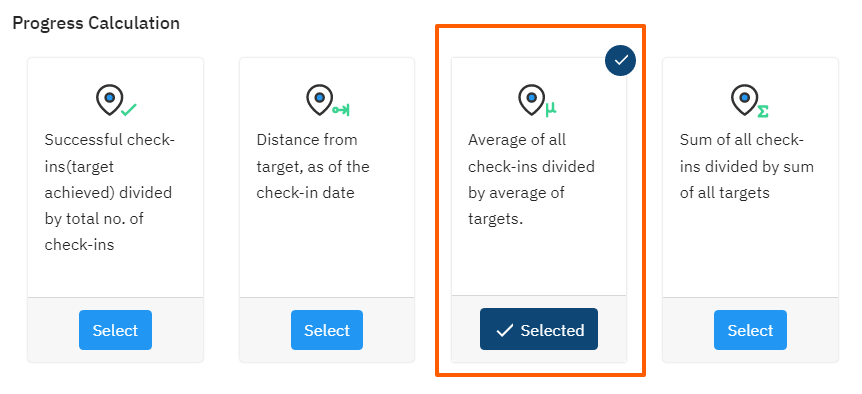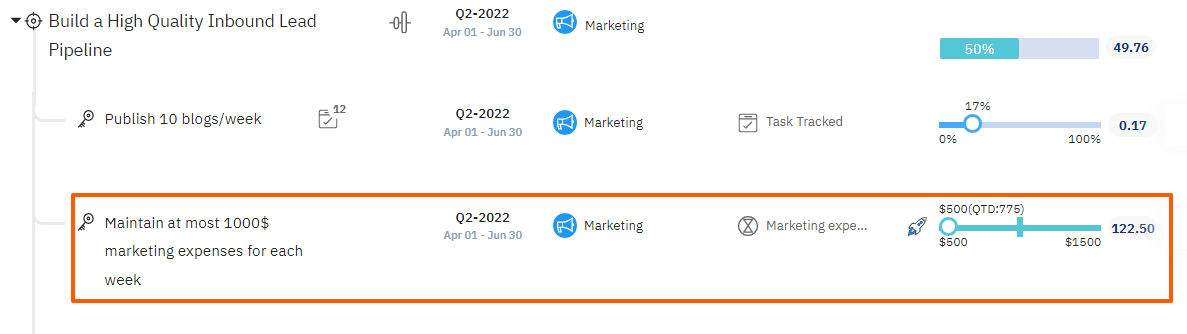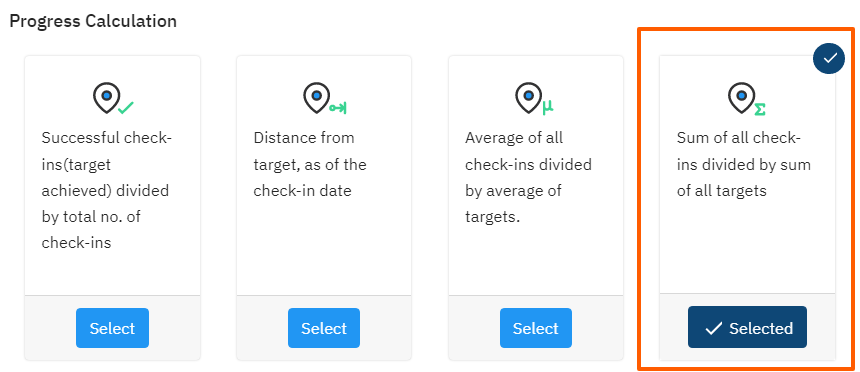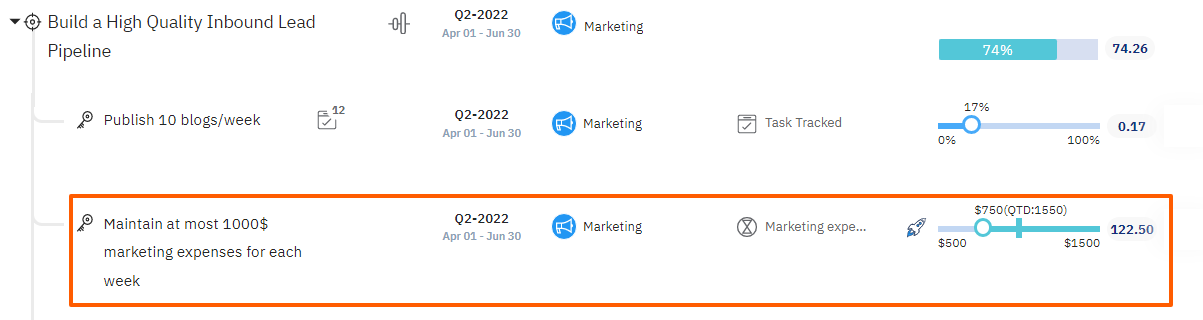# How do I calculate the Average/Sum cumulative value for the Control KPI Key Result?

Category: KPIs

Profit.co has progress calculation methods for the control KPI key results. Average of all check-ins divided by the average of targets & Sum of all check-ins divided by the sum of all targets

Why this feature?

By using this feature the key result can be progressed based on the check-in value’s Average/Sum of the target and the cumulative value of all check-ins, The calculation will be displayed in the slider bubble as QTD/ YTD value.

Average of all check-ins divided by average of targetsIn this type of progression, the average of all the check-ins will be taken into consideration whether the check-in is successful or unsuccessful. The Progress will happen and the final calculation will be based on the average.

Additionally based on the target date we have QTD/YTD value in the check-in slider. If the key result is in the Quarter period, It will be mentioned as QTD. If it is an Annual period, It will be mentioned as YTD.

The Average of all the check-ins will be taken as QTD/ YTD value in the check-in slider.

Then the progress achievement score will be calculated with QTD value based on the below formula for progress calculation:

The progress Calculation formula is,

>100, 100+(diff/target*100)

For example, We have a Key result to Maintain at most \$1000 marketing expenses for each week

First check-in 800

Second check-in 750

Average = 775

Here 1000 is Average of Target value(1000+ 1000)/2

Difference = 1000-775 = 225

Progress = 100+ 225/1000 = 100+22.5 =122.5%

Progress = 122.5%

QTD = \$775Sum of all check-ins divided by sum of all targets.In this type of progression, An unsuccessful check-in will also be taken into account and the progress will be shown in the Sum. The sum of all the check-ins will be taken into consideration whether the check-in is successful or unsuccessful.

The Sum of all the check-ins will be taken as QTD/ YTD value in the check-in slider.

Progress achievement scores will be calculated with the QTD value.

The progress calculation formula

>100, 100+(diff/target*100)

For example, We have a Key result to Maintain at most \$1000 marketing expenses for each week

First check-in 800

Second check-in 750

Sum = 1550

Target sum =2000

Here Target value is 2000 i.e, Sum of target value(2 x 1000)

Difference = 2000-1550 = 450

Progress = 100+ 450/2000 = 100+22.5 =122.5%

Progress = 122.5%

QTD = 1550#### Related Questions

• ##### What is Aggregation Level?

Aggregation level allows you to define the depth of aggregation. There are 2 levels available to choose from: Aggregate from entire tree of contributing OKRs Aggregate from only the next level of contributing OKRs ...Read more

• ##### What is KPI Extension?

While KPI aggregation works for the same KPI, KPI Extension can get aggregated value up to the extended KPI from ...Read more

• ##### How do you authorize the connector in salesforce?

Profit.co provides an option to enable the connector that helps users to access Salesforce. Users can create, edit and ...Read more

• ##### How to create a KPI and connect it with a Key Result via a connector?

Profit.co provides an option to create a new KPI and connect it with a Key Result via a connector to automate the key ...Read more